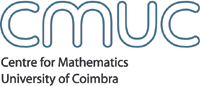AnalysisMembersSeminarsEventsPublicationsdetails Title Algebraic and continuous properties of multilinear forms AbstractLet $X$ be a vector space and let $\phi, \psi \in X^\ast$ be two linear forms on $X.$ It is well known that if, for any $x \in X,$ $$\phi(x) = 0 \Rightarrow \psi(x) = 0,$$ then $\psi = c \phi$ for some scalar $c.$ A continuous version is also known. Namely, if $X$ has a norm, if $||\phi|| = \| \psi \| = 1,$ and if, for any $x \in X,$ with $\|x\| = 1,$ $$\phi(x) = 0 \Rightarrow |\psi(x)| < \epsilon,$$ then either $||\phi + \psi|| < 2\epsilon$ or $||\phi - \psi|| < 2\epsilon.$ In this talk, we will discuss analogous questions for the case of multilinear forms on a product of spaces, $X_1 \times \cdots \times X_n \to \mathbb{K}.$ (This is joint work with A Cardwell, L. Downey, D. García, M. Maestre, and I. Zalduendo.) Speaker(s) Richard M. Aron (Kent State University, USA) Date June 03, 2005Time14.30 Room 5.5 CMUC Apartado 3008, 3001 - 454 Coimbra, Portugal T:+351 239 791 150 F:+351 239 793 069 cmuc@mat.uc.pt - developed by Flor de Utopia